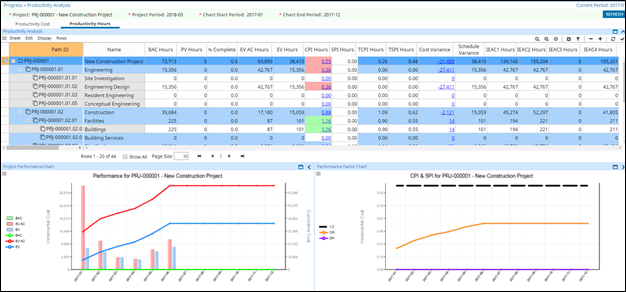Productivity Analysis Dashboard - EcoSys - 3.01 - Help - Hexagon PPM

EcoSys Projects Help (3.01)

Language
English (United States)
Product
EcoSys
Search by Category
Help
EcoSys Standard Version
3.01

The Productivity Analysis Dashboard displays a variety of earned value costs and metrics. Charts portray earned value data in a graphical format. Drilldown functionality provides additional detail.

Data may be viewed by cost or by units.1. Navigate to Progress > Productivity Analysis. The Project Budget Status and the Project Budget Status Chart panels appear.

2. At the top of the screen, in the green parameter bar:

1. Click the Project ellipsis to select the required project.

2. Click the Project Period ellipsis to select the required project period.

3. Click the Chart Start Period ellipsis to select the required start date in the chart.

4. Click the Chart End Period ellipsis to select the required end date in the chart.

3. Click Refresh for screen update.

4. Click the Productivity Cost tab. The productivity analysis costs appear in the Productivity Analysis panel and the Project Performance Chart and Performance Factor Charts appear. To view the charts related to productivity costs,

1. View the project performance cost chart in the Project Performance Chart panel. The BAC (Budget At Completion), EV AC (Earned Value Actuals), and EV (Earned Value) values appear in a bar chart format.

2. To view the performance variance chart or performance factor chart for specific object, perform any of the following:

• Click the CPI Cost hyperlink. The CPI and SPI costs specific to the object appear in a bar chart format.

• Click the Cost Variance hyperlink. The cost variance and schedule variance specific to the object appear in a bar chart format.

The below listed details appear in the Productivity Analysis panel:

• Path ID: Displays the path ID of the object.

• Name: Displays the name of the of the object.

• BAC Cost: Displays the budget at completion value of the object.

• PV Cost: Displays the present value cost of the object.

• % Complete: Displays the percentage complete of the object.

• EV AC Cost: Displays the sum of estimated actuals costs and actuals costs of the object.

• EV Cost: Displays the earned value cost of the object.

• CPI Cost: Displays the cost performance index value of the object.

• SPI Cost: Displays the schedule performance index value of the object.

• TCPI Cost: Displays to complete performance index value of the object.

• TSPI Cost: Displays to complete schedule performance indicator value of the object.

• Cost Variance: Displays the cost variance of the object.

• Schedule Variance: Displays the schedule variance of the object.

• IEAC1 Cost: Displays the independent estimate at completion of the object. The formula used to calculate is BAC/CPI.

• IEAC2 Cost: Displays the independent estimate at completion of the object. The formula used to calculate is AC + (BAC – EV/ (CPI*.8) + (SPU*.2).

• IEAC3 Cost: Displays the independent estimate at completion of the object. The formula used to calculate is AC + (BAC-EV/CPI*SPI).

• IEAC4 Cost: Displays the independent estimate at completion of the object. The formula used to calculate is AC + (BAC-EV).

5. Click the Productivity Hours tab. The productivity analysis costs appear in the Productivity Hours panel and the Project Performance Chart and Performance Variance Charts appear. To view the charts related to productivity hours,

1. View the project performance hours chart in the Project Performance Chart panel. The BAC, EV AC, and EV values appear in a bar chart format.

2. To view the performance variance chart or performance factor chart for specific objects, perform any of the following:

• Click the CPI Cost hyperlink. The CPI and SPI costs specific to the object appear in a bar chart format.

• Click the Cost Variance hyperlink. The cost variance and schedule variance specific to the object appear in a bar chart format.

The below listed details appear in the Productivity Hours panel:

• Path ID: Displays the path ID of the object.

• Name: Displays the name of the of the object.

• BAC Hours: Displays the budget at completion hours of the object.

• PV Hours: Displays the present value hours of the object.

• % Complete: Displays the percentage complete of the object.

• EV AC Cost: Displays the sum of estimated actuals and actuals hours of the object.

• EV Hours: Displays the earned value hours of the object.

• CPI Hours: Displays the cost performance index hours of the object.

• SPI Hours: Displays the schedule performance index hours of the object.

• TCPI Hours: Displays to complete performance index hours of the object.

• TSPI Hours: Displays to complete schedule performance indicator hours of the object.

• Cost Variance: Displays the cost variance of the object.

• Schedule Variance: Displays the schedule variance of the object row.

• IEAC1 Hours: Displays the independent estimate at completion of the object. The formula is BAC/CPI.

• IEAC2 Hours: Displays the independent estimate at completion of the object. The formula used to calculate IEAC2 Cost is AC + (BAC – EV/ (CPI*.8) + (SPU*.2).

• IEAC3 Hours: Displays the independent estimate at completion of the object. The formula is AC + (BAC-EV/CPI*SPI).

• IEAC4 Hours: Displays the independent estimate at completion of the object. The formula is AC + (BAC-EV).